• 线性代数
• 概率论与数理统计
• 信息论基础

## 线性代数

### Scalar, Vectors, Matrices, Tensors的概念

Scalar: 标量，可以看成一个数
Vector: 向量，可以看成一个一维数组
Matrix: 矩阵，可以看成二维数组
Tensor: 张量，三维或三维以上的数组的统称，维度不定

### 范数(Norm)

$L^p$范数定义为：
$$||x||_p=(\Sigma_i {|x_i|^p})^{\frac 1 p}$$

$x^{\top}x=||x||_2^2$

Frobenius Norms: $||A||_{F}=\sqrt{\Sigma_{i,j}A_{i,j}^2}$

### 特殊矩阵

（1）A的特征值为实数，且其特征向量为实向量
（2）A的不同特征值对应的特征向量必定正交
（3）A一定有n个线性无关的特征向量，从而A相似于对角矩阵

### 奇异值分解(Singular Value Decomposition)

$A=UDV^{-1}$，U称为左奇异向量，为$AA^{\top}$的特征向量，V称为右奇异向量，为$A^{\top}A$的特征向量。

### Moore-Penrose Pseudoinverse

(1) $AXA = A$
(2) $XAX = X$
(3) $(AH)^H = AX$
(4) $(XA)^H = XA$
（$A^H$为共轭转置，即$\bar{A} ^ T$）

### 迹运算符(Trace)

$Tr(A) = {\Sigma}_i A_{i,i}$

## 概率论

• 系统固有的随机性
比如说打牌的游戏，我们都会假设洗牌确实是能够将牌的顺序变的随机。
• 不完备的观察
即使是一个确定的系统，当我们不能够观察到系统中所有驱动系统行为的变量时，也是会呈现出随机性的。
• 不完备的建模
我们有时候建模时无法完美地使用所有信息，从而必须丢弃一些观察到的信息，丢弃的信息就会造成模型预测的不确定性。

### 概率分布

• 离散随机变量的概率分布称为概率质量函数(PMF, Probablity Mass Function)
• 连续随机变量的概率分布称为概率密度函数(PDF, Probablity Density Function)

### 边缘概率

$$\forall x \in X, P(X=x) = \sum_y {P(X=x, Y=y)}$$

$$p(x)=\int p(x,y)dy$$

### 条件概率及其链式法则

$$P(Y=y | X=x) = \frac {P(Y=y,X=x)} {P(X=x)} \ \ \ \ \ \ \ \ \ \ (P(X=x) > 0)$$

$$P(x^{(1)}, x^{(2)}, …, x^{(n)}) = P(x^{(1)})\prod_{i=2}^n P(x^{(i)} | x^{(1)}, x^{(2)}, …, x^{(i-1)})$$

### 独立和条件独立

$$\forall x \in X, y \in Y, p(X=x, Y=y)=P(X=x)P(Y=y)$$

$$\forall x \in X, y \in Y, z \in Z, p(X=x, Y=y | Z=z)=P(X=x | Z=z)P(Y=y | Z=z)$$

### 期望，方差与协方差

$$\mathbb{E}[f(x)] = \sum_x P(x)f(x)$$

$$\mathbb{E}[f(x)] = \int p(x)f(x)dx$$

$$E(X)=\sum_{x}|f(x)|P(x) < \infty$$

$$Var(X) = \mathbb{E}[(X-\mathbb{E}[X])^2]$$

$$Cov(X,Y) = \mathbb{E}[(X-EX)(Y-EY)]$$

$$Cov({\bf{x}})_{i,j} = Cov(x_i, x_j)$$

### 常用概率分布

• 贝努利分布
• 多贝努利分布/多类分布
• 高斯分布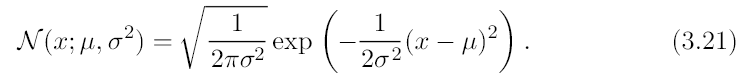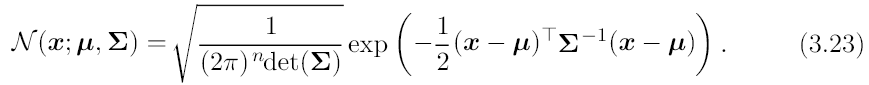• 指数分布
• 拉普拉斯分布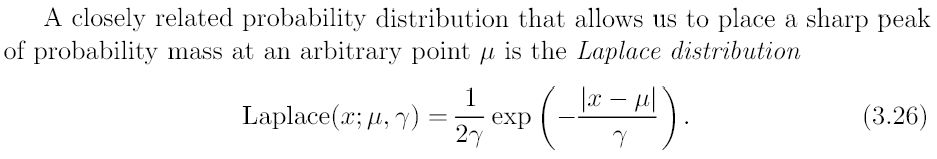• 狄拉克分布(Dirac)与经验分布
• 混合分布

### 一些有用的性质和通用函数

Sigmoid函数：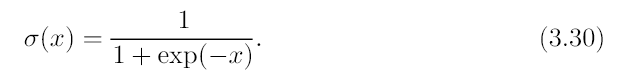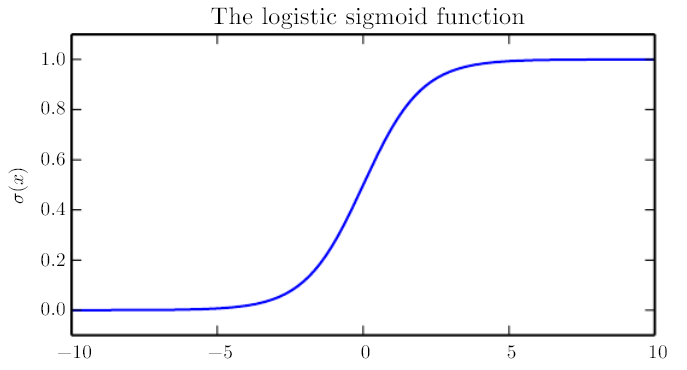Softplus函数：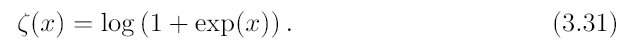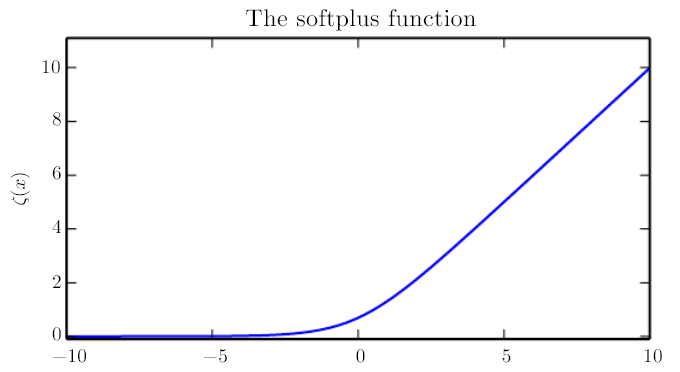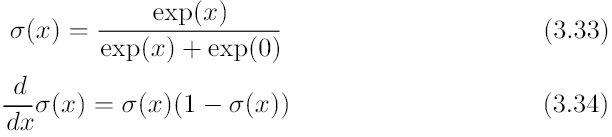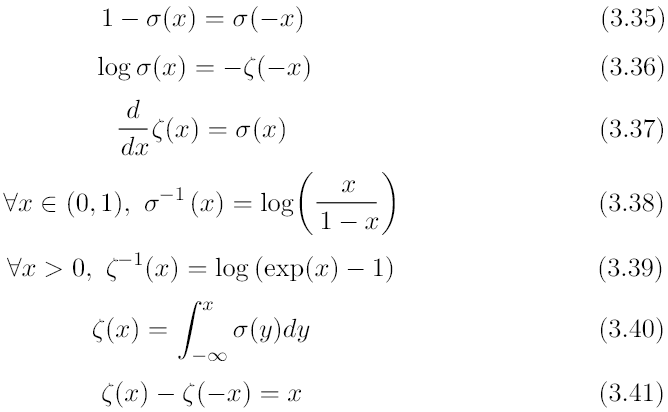### 贝叶斯规则

Reverend Thomas Bayes发明了贝叶斯公式，现在一般写成：
$$P(x | y) = \frac {P(x)P(y | x)} {P(y)}$$
$P(y)$如果不知道的话，可以以$P(y)=\sum_x P(y|x)P(x)$计算。

## 信息论

• 非常可能的事件信息量比较少，更不可能发生的事件具有更高的信息量
• 独立事件应具有增量的信息。比如投两次硬币正面向上的信息，应该是投掷一次正面朝上的信息量的2倍。

$$H(x) = \mathbb{E}_{x - P}[I(x)] = -\mathbb{E}_{x - P}[\log P(x)]$$

KL散度

$$D_{KL}(P||Q) = \mathbb{E}_{x - P}[\log {\frac {P(x)} {Q(x)}}] = \mathbb{E}_{x - P}[\log P(x) - \log Q(x)]$$

KL散度非负。在离散型变量情况下，KL散度为0当且仅当P,Q为相同分布。在连续型变量情况下，KL散度为0当且仅当P,Q“几乎处处相同”。

$$H(P,Q) = -\mathbb{E}_{x - P} {\log Q(x)}$$